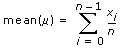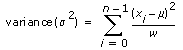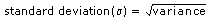StandardDeviation Method

Measurement Studio 2012 for Visual Studio 2010 Help

Edition Date: August 2012

Part Number: 372636F-01

»View Product InfoDownload Help (Windows Only)

Computes the standard deviation of the values in the input array.

Namespace: NationalInstruments.Analysis.Math

Assembly: NationalInstruments.Analysis.Enterprise (in NationalInstruments.Analysis.Enterprise.dll) Version: 12.0.40.318

Syntax

Visual Basic (Declaration)
<SecurityPermissionAttribute(SecurityAction.Demand, Flags := SecurityPermissionFlag.UnmanagedCode)> _
Public Shared Function StandardDeviation ( _
inputData As Double() _
) As Double

C#
[SecurityPermissionAttribute(SecurityAction.Demand, Flags = SecurityPermissionFlag.UnmanagedCode)]
public static double StandardDeviation(
double[] inputData
)

Parameters

inputData
Type: System.Double []
The input array.

Return Value

The calculated standard deviation of the values in inputData.

Exceptions

ExceptionCondition
ArgumentException The number of samples in inputData is equal to zero.
ArgumentNullException inputData is null (Nothing in Visual Basic).
DllNotFoundException The analysis library cannot be found.
EntryPointNotFoundException A required operation in the analysis library cannot be found.

Remarks

This method computes the population standard deviation of the input data. To determine the sample standard deviation, multiply the value returned by this function by the result of The values are computed using the following formulas:where n represents the number of elements in X, and
X represents inputData.where w represents n for population and (n–1) for sample.To determine the sample standard deviation, multiply the value returned by this function by the result ofExamples

The following example calculates the standard deviation of the generated noise signal.
VB.NET
Dim inputData(1999) As Double
Dim standardDeviation As Double
Dim gaussianNoise As GaussianNoiseSignal = New GaussianNoiseSignal(1.0, 17)

' Generate a Gaussian noise signal that represents inputData
inputData = gaussianNoise.Generate(1000.0, 200)

standardDeviation = Statistics.StandardDeviation(inputData)
C#
double[] inputData = new double;
double standardDeviation;
GaussianNoiseSignal gaussianNoise = new GaussianNoiseSignal(1.0, 17);

// Generate a Gaussian noise signal that represents inputData
inputData = gaussianNoise.Generate(1000.0, 2000);

standardDeviation = Statistics.StandardDeviation(inputData);